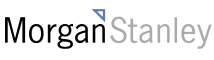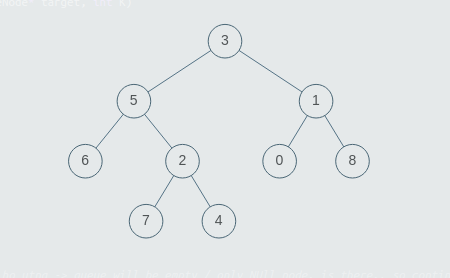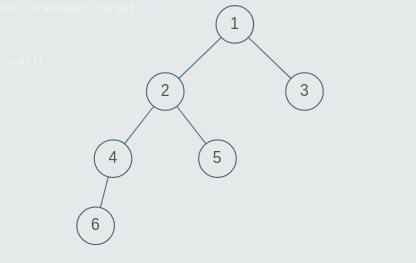New update is available. Click here to update.
Topics

# Left Sum

Easy0/40
Average time to solve is 10m## Problem statement

Given a binary tree having ‘N’ number of nodes. Your task is to find the sum of all left nodes present in the input binary tree. That is, you need to take the sum of all nodes which are the left child of some node.

Note :

``````1. A binary tree is a tree in which each node can have at most two children.
2. The given tree will be non-empty i.e the number of non-NULL nodes will always be greater than or equal to 1.
3. Multiple nodes in the tree can have the same values, all values in the tree will be positive.
``````
Detailed explanation ( Input/output format, Notes, Images )
Constraints:
``````1 <= ‘T’ <= 5
1 <= ‘N’ <= 3 * (10 ^ 3)
1 <= ‘nodeVal’ <= 10 ^ 9
Where ‘N’ represents the number of nodes in a binary tree,
‘nodeVal’ represents the value of a node.

Time Limit: 1 sec.
``````
Sample Input 1 :
``````2
3 5 1 6 2 0 8 -1 -1 7 4 -1 -1 -1 -1 -1 -1 -1 -1
1 2 3 4 5 -1 -1 6 -1 -1 -1 -1 -1
``````
Sample Output 1 :
``````18
12
``````
Explanation for Sample Input 1 :``````Test Case 1 :
The nodes 5, 6, 7, and 0 are the only nodes that were the left child of any other node. S, they sum up to make the sum 18.
````````````Test Case 2 :
The nodes 2, 4, and 6 are the only nodes that were the left child of any other node. S, they sum up to make the sum 12.
``````
Sample Input 2 :
``````2
5 2 3 8 1 -1 -1 7 9 -1 -1 5 6 -1 -1 -1 -1 -1 -1
1 5 7 -1 -1 6 3 9 8 -1 -1 -1 -1 13 -1 -1 -1
``````
Sample Output 2 :
``````22
33
``````Console